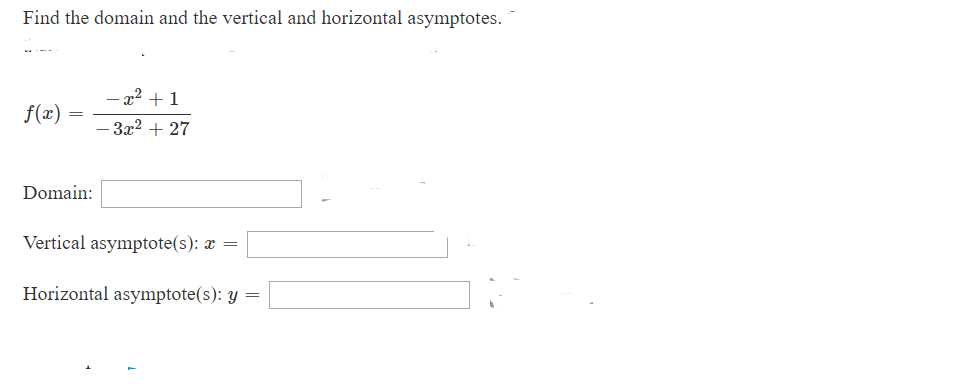# Find the domain and the vertical and horizontal asymptotes-2 1f(x)- 3x2 + 27DomainVertical asymptote(s): æ=Horizontal asymptote(s): y =

Question
2 viewshelp_outlineImage TranscriptioncloseFind the domain and the vertical and horizontal asymptotes -2 1 f(x) - 3x2 + 27 Domain Vertical asymptote(s): æ = Horizontal asymptote(s): y = fullscreen
check_circle

Step 1

Part (a):

Domain of f (x) = (- x2 + 1) / (-3 x2 + 27)

The domain of the function is the set of input for which function is real and defined.

Take the denominator and compare to zero

Solve – 3 x2 + 27 = 0 given x = 3 and x = -3

The point x = 3 and x = -3 are undefined. Hence the domain of the function is x < - 3 0r -3 < x < 3 or x > 3.

Step 2

Part (B)

For rational function, the vertical asymptotes are the undefined points, also known as the zeros of the denominator, of the simplifies function. The undefined points are x = 3 and x = -3.

Hence, the vertical asymptotes are x = 3 and x = -3.

Step 3

Part (c)

If denominator’s degree > numerator’s degree, the horizontal asymptotes is the

X – axis: y = 0.

If the degree is equal, the asymptote is y = numerator’s leading coefficient / denominators leading coefficient.

Here in the function:

The degree of the denominator = 2. The degree of the numerator is 2.

&n...

### Want to see the full answer?

See Solution

#### Want to see this answer and more?

Solutions are written by subject experts who are available 24/7. Questions are typically answered within 1 hour.*

See Solution
*Response times may vary by subject and question.
Tagged in

### Other